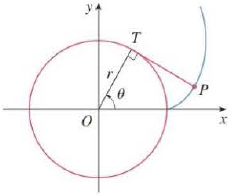Chapter 10.2, Problem 73E

Chapter
Section
Textbook Problem

# A string is wound around a circle and then unwound while being held taut. The curve traced by the point P at the end of the siring is called the involute of the circle. If the circle has radius r and center O and the initial position of P is (r, 0), and if the parameter θ is chosen as in the figure, show that parametric equations of the involute are x = r ( cos θ + sin θ ) y = r ( sin θ − θ cos θ )To determine

To find: The parametric equations of the involute shape traced by the string which is wound around a circle.

Explanation

Given:

Initial position of point P for the involute is (r,0) and angle θ related to position with respect to time is taken as shown below in the Figure 1.

The radius of the circle is denoted as r. The center of the circle is denoted as O.

Refer to the above figure 1. Extend the line OT till some finite point R. Draw a line parallel to x axis from point T till point Q, where it will intersect the involute curved traced by the string. The inclination of line OT with respect to horizontal x axis is θ and this line will move and change with time.

The figure 2 illustrates the points mentioned above in it.

Refer the figure 2, identify the various angles as below.

RTQ=θOTP=π2RTP=π2QTP=RTPRTQ=π2θ

The parametric equation of a circle for the variable x is as below.

x=rcosθ

The parametric equation of a circle for the variable y is as below.

y=rsinθ

Analyze the start of involute, when the string is just about to be unwound from the initial Position of P, with coordinate (r,0).

The coordinate of T is (rcosθ,rsinθ).

The length of side TP is given by |TP|=r×θ.

Here, r is the radius of the circle, and θ is the angle at the center of the circle.

Consider the triangle ΔQTP

### Still sussing out bartleby?

Check out a sample textbook solution.

See a sample solution

#### The Solution to Your Study Problems

Bartleby provides explanations to thousands of textbook problems written by our experts, many with advanced degrees!

Get Started